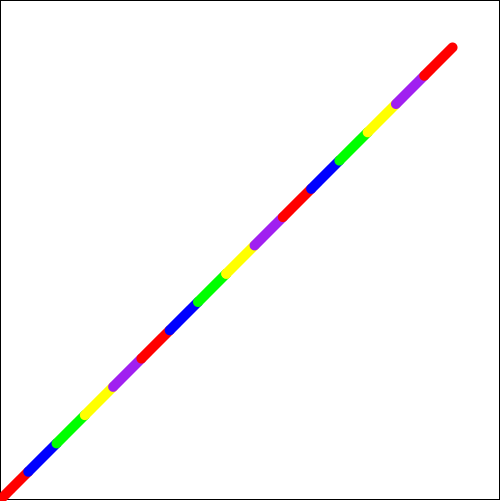8.3

4Racket Turtle examples

4.1Drawing a square

First you need to define a list of turtle commands for drawing a square. We will name it square1. Actual drawing of the turtle image is done using draw function, the turtle command list is given as an argument to draw.

 (define square1 (list (forward 100) (turn-left 90) (forward 100) (turn-left 90) (forward 100) (turn-left 90) (forward 100)))

(draw square1)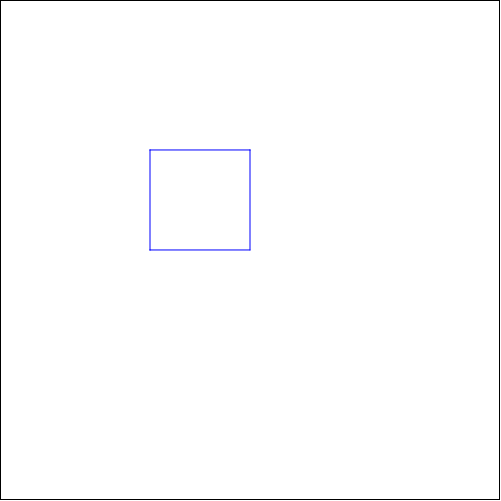4.2Drawing a square using repeat

Since the command list for drawing a square has a repeating pattern, it is better to define it separately and use it repeatedly. We will name it side and we will repeat it 4 times using repeat.

 (define side (list (forward 100) (turn-left 90)))
 (define repeat-square (repeat 4 side))

(draw repeat-square)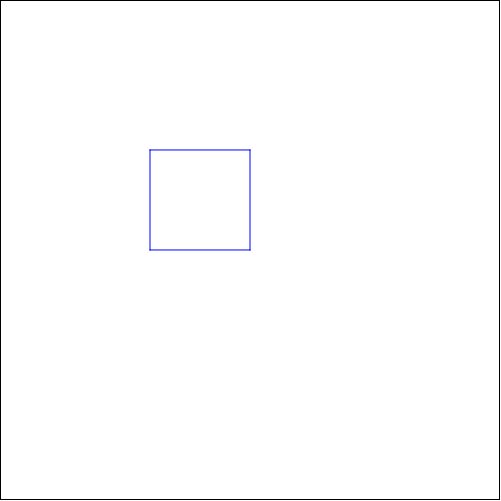4.3Drawing two squares in a same picture

We can use a previously defined square and draw two of those in the same picture. We will define a list of commands move to move to a new location and changes the pen color before drawing the second square.

 (define move (list (pen-up) (turn-right 90) (forward 100) (pen-down) (change-color "red")))
 (define two-squares (list square1 move square1))

(draw two-squares)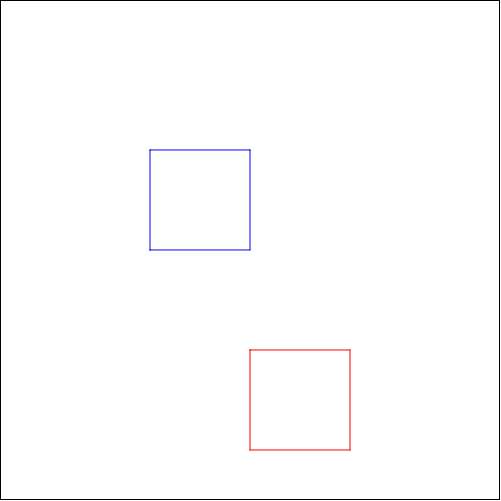4.4Drawing a square using a function

To be able to draw squares with changing side lengths, we need to define a function, which we will name changing-square. We need also a helper function changing-side to draw sides with changing lengths. The variable x is the side length. Finally we call the new function changing-square with argument 30 (x=30).

 (define (changing-side x) (list (forward x) (turn-left 90)))
 (define (changing-square x) (repeat 4 (changing-side x)))

(draw (changing-square 30))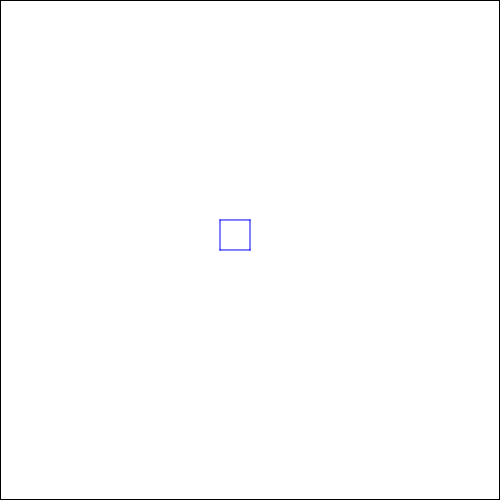4.5Drawing a square using coordinates

You can draw a square also by ordering the turtle to go via some points in the coordinate plane using go-to commands. To get easier coordinates we change the place of the origin with set-origin command.

 (define coordinate-square (list (set-origin) (go-to 100 0) (go-to 100 100) (go-to 0 100) (go-to 0 0)))

(draw coordinate-square)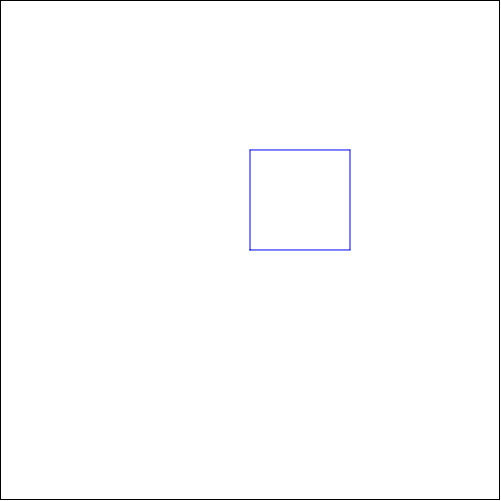4.6Mirroring a square

We can draw two square so that the second one is created by mirroring the first one using a pivot point. The pivot point is the location where the mirroring was turned on (mirron-x-on, mirron-y-on), in this example it is the starting point.

 (define mirroring-square (list (mirror-x-on) (mirror-y-on) square1))

(draw mirroring-square)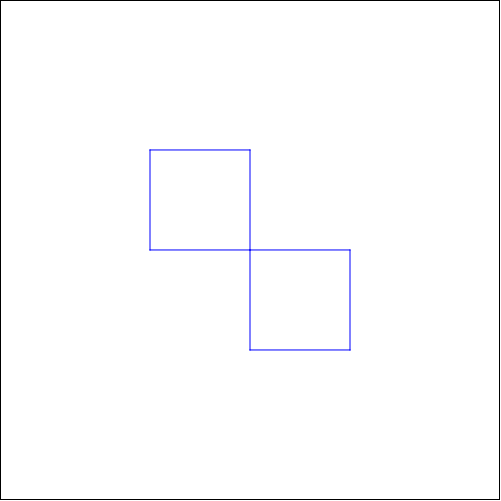4.7Drawing a square using a stamper

We can activate the stamper functionality using stamper-on command. Now the turtle will "stamp" the given image after each movement. Here we use a red circle as the stamp. You could also let the pen draw the line also (here is it taken up).

(define STAMP (circle 5 "solid" "red"))

 (define stamper-square (list (stamper-on STAMP) (pen-up) square1))

(draw stamper-square)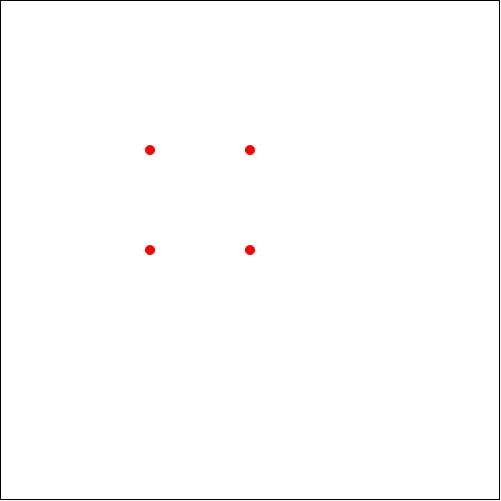4.8Changing pen style and size

You can change the style of the line using change-pen-style command and the width of the line using change-pen-size command.

Note! This doesn’t work in WeScheme.

 (define special-pen-square (list (change-pen-size 5) (change-pen-style "dot") square1))

(draw speacial-pen-square)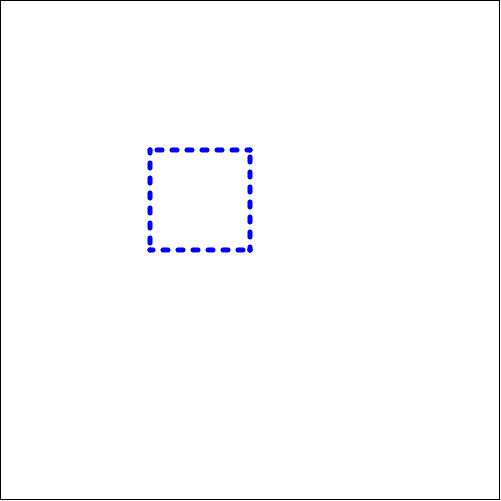4.9Drawing a line in a grid

First we draw a background grid using set-bg-grid. We move to the origin using (go-to 0 0) and activate the stamper with a blue circle as the stamp. To get the stamps in the right coordinates we need to command the turtle using go-to multiple times. In this example the stamper is inactivated after four points .

 (define line-with-grid (list (set-bg-grid 20 20 "pink") (pen-up) (go-to 0 0) (stamper-on (circle 5 "solid" "blue")) (pen-down) (go-to 40 40) (go-to 80 80) (go-to 120 120) (go-to 160 160) (stamper-off) (go-to 500 500)))

(draw line-with-grid)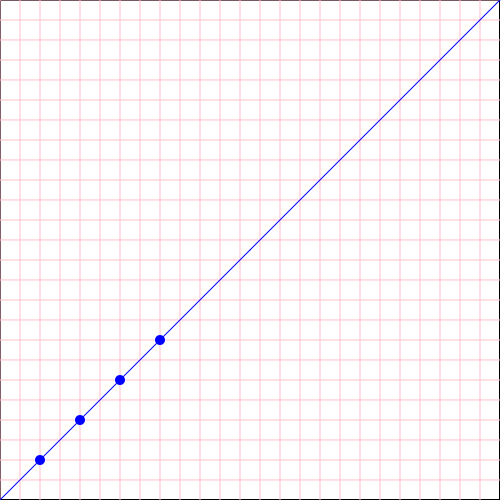4.10Drawing a line with multiple stamps

We move to origin using go-to-origin command and draw a line using a list of stamps.

 (define STAMPS (list (circle 10 "solid" "red") (star 10 "solid" "blue") (circle 10 "solid" "green") (star 10 "solid" "yellow") (circle 10 "solid" "black")))
 (define line-with-stamps (list (pen-up) (go-to-origin) (turn-right 45) (stamper-on STAMPS) (repeat 8 (forward 50))))

(draw line-with-stamps)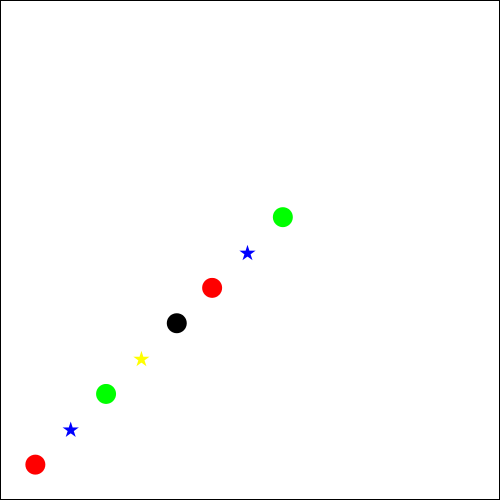4.11Changing background color, background image and animation size

You can change the background color using change-bg-color color and you can put an additional image on top of it using set-bg-image. In the example we want to draw a picture with different dimensions so draw-custom is used (setting the drawing speed to zero will not affect the drawing speed).

 (define square-over-bg (list (change-bg-color "black") (set-bg-image (circle 100 "solid" "gold")) square1))

(draw square-over-bg 400 250 0)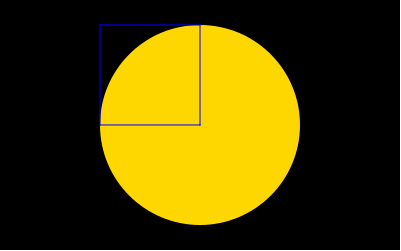4.12A line with changing colors

In this example we set the pen color to be a list of colors. Turtle will use each color one after another. The animation speed has been set to be slower (the last argument of draw-custom). The turtle is also hidden during the first part of the animation.

 (define COLORS (list "red" "blue" "green" "yellow" "purple"))
 (define color-line (list (pen-up) (go-to 0 0) (turn-right 45) (pen-down) (change-color COLORS) (change-pen-size 10) (hide-turtle) (repeat 8 (forward 40)) (show-turtle) (repeat 8 (forward 40))))

(draw-custom color-line 500 500 0.5)